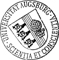## Adaptive Finite Elements for Optimally Controlled Elliptic Variational Inequalities of Obstacle Type

• We are concerned with the numerical solution of distributed optimal control problems for second order elliptic variational inequalities by adaptive finite element methods. Both the continuous problem as well as its finite element approximations represent subclasses of Mathematical Programs with Equilibrium Constraints (MPECs) for which the optimality conditions are stated by means of stationarity concepts in function space  and in a discrete, finite dimensional setting  such as (ɛ-almost, almost) C- and S-stationarity. With regard to adaptive mesh refinement, in contrast to the work in  which adopts a goal oriented dual weighted approach, we consider standard residual-type a posteriori error estimators. The first main result states that for a sequence of discrete C-stationary points there exists a subsequence converging to an almost C-stationary point, provided the associated sequence of nested finite element spaces is limit dense in its continuous counterpart. As theWe are concerned with the numerical solution of distributed optimal control problems for second order elliptic variational inequalities by adaptive finite element methods. Both the continuous problem as well as its finite element approximations represent subclasses of Mathematical Programs with Equilibrium Constraints (MPECs) for which the optimality conditions are stated by means of stationarity concepts in function space  and in a discrete, finite dimensional setting  such as (ɛ-almost, almost) C- and S-stationarity. With regard to adaptive mesh refinement, in contrast to the work in  which adopts a goal oriented dual weighted approach, we consider standard residual-type a posteriori error estimators. The first main result states that for a sequence of discrete C-stationary points there exists a subsequence converging to an almost C-stationary point, provided the associated sequence of nested finite element spaces is limit dense in its continuous counterpart. As the second main result, we prove the reliability and efficiency of the residual-type a posteriori error estimators. Particular emphasis is put on the approximation of the reliability and efficiency related consistency errors by heuristically motivated computable quantities and on the approximation of the continuous active, strongly active, and inactive sets by their discrete counterparts. A detailed documentation of numerical results for two representative test examples illustrates the performance of the adaptive approach.Author: Alexandra GaevskayaGND, Michael HintermüllerGND, Ronald H. W. HoppeGND urn:nbn:de:bvb:384-opus4-24417 https://opus.bibliothek.uni-augsburg.de/opus4/2441 Preprints des Instituts für Mathematik der Universität Augsburg (2013-15) Preprint English Universität Augsburg 2013/08/14 optimal control; elliptic variational inequalities; stationarity; finite elements; a posteriori error analysis Optimale Kontrolle; Elliptische Variationsungleichung; Fehlerabschätzung; A-posteriori-Abschätzung; Finite-Elemente-Methode Mathematisch-Naturwissenschaftlich-Technische Fakultät Mathematisch-Naturwissenschaftlich-Technische Fakultät / Institut für Mathematik Mathematisch-Naturwissenschaftlich-Technische Fakultät / Institut für Mathematik / Lehrstuhl für Numerische Mathematik 5 Naturwissenschaften und Mathematik / 51 Mathematik / 510 MathematikDeutsches Urheberrecht mit Print on Demand